# Texas Go Math Grade 1 Lesson 1.1 Answer Key Understand Ten and Ones

Refer to our Texas Go Math Grade 1 Answer Key Pdf to score good marks in the exams. Test yourself by practicing the problems from Texas Go Math Grade 1 Lesson 1.1 Answer Key Understand Ten and Ones.

## Texas Go Math Grade 1 Lesson 1.1 Answer Key Understand Ten and Ones

Essential Question
How can you use different ways to write a number as ten and ones?
Explanation:
By making to 10 frame
13 in 3 different ways
1 tens 3 ones
10 + 3 = 13
10 ones 3 ones

Explore
Use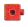to model the problem.
Draw theto show your work.For The Teacher

• Read the problem. Tim has 10 pennies. He gets 2 more pennies. How many pennies does Tim have now?
Explanation:Tim has 10 pennies. He gets 2 more pennies.
10 + 2 = 12
I tens and 2 ones
12 pennies that Tim have now

Math Talk
Mathematical processes
How does your picture show the pennies Tim has? Explain.
Explanation:
Tim has 10 pennies. He gets 2 more pennies.
10 + 2 = 12
I tens and 2 ones
12 pennies that Tim have now

Model and Draw

13 is a two-digit number. The 1 in 13 means 1 ten. The 3 in 13 means 3 ones.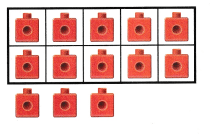Think
10 ones and 3 ones is the same as 1 ten 3 ones.
______ ten ______ ones
____ + _____
_____
Explanation:
1 ten 3 ones
10 + 3
13
10 ones = 1 tens.

Share and Show

Use the model. Write the number three different ways.

Question 1.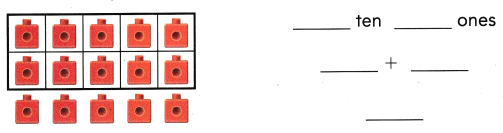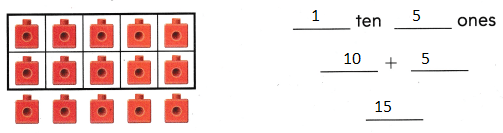Explanation:
10 + 5 = 15
1 tens and 5 ones
10 ones and 5 ones
This shows the 3 different ways
The sum of 10 and 5 is 15

Question 2.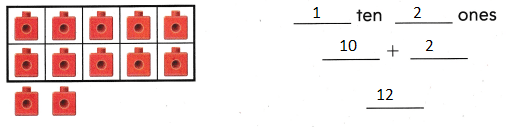Explanation:
10 + 2 = 12
1 tens and 2 ones
10 ones and 2 ones
This shows the 3 different ways
The sum of 10 and 2 is 12

Problem Solving

Use the model. Write the number three different ways.

Question 3.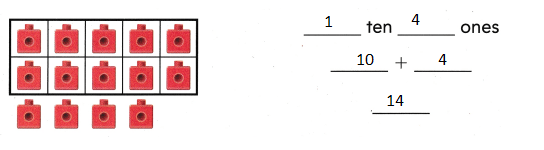Explanation:
10 + 4 = 14
The sum of 10 and 4 is 14

Draw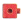to show the number. Write the number three different ways.

Question 4.
H.O.T. Karen has 7 ones. Jimmy has 9 ones. They put all their ones together. What number did they make?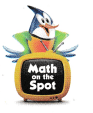Explanation:
7 + 9 = 16
1 tens and 6 ones
10 ones and 6 ones
we can write in these 3 ways

Question 5.
H.O.T. Multi-Step Emma has 5 ones. Caden has 3 ones. They put all their ones together. Alex put 1 ten with the ones. What number did they make?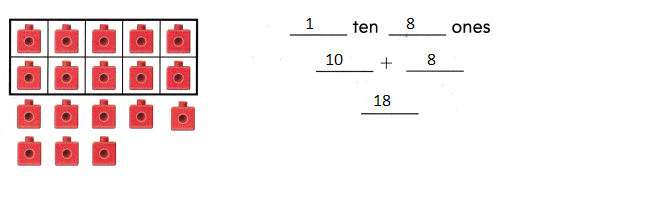Explanation:
5 + 3 = 8
10 + 8 = 18
1 tens and 8 ones

Question 6.
Apply Two friends tossed 18 juggling balls back and forth 12 times. Which shows 18?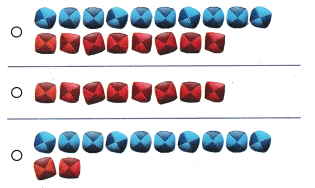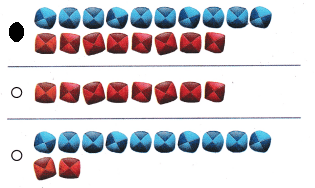Explanation:
1 tens and 8 ones
Bubbled that shows 18

Question 7.
Multi-Step Mona has 1 ten and 3 ones. Then Nate and Avery each gave 2 ones to Mona. How many tens and ones does Mona have now?
(A) 1 ten 0 ones
(B) 1 ten 7 ones
(C) 7 tens 1 one
Explanation:
1 tens and 7 ones does Mona have now
3 + 2 + 2 = 7
1 ten 7 ones

Question 8.
Texas Test Prep Which shows the same number?(A) 1 ten 12 ones
(B) 1 ten 2 ones
(A) 1 ten 7 ones
Explanation:
1 ten 2 ones shows the same number
10 + 2 = 12

Take Home Activity

• Show your child one group of 10 pennies and one group of 8 pennies Ask your child to tell how many tens and ones there are, and say the number. Repeat with other numbers from 11 to 19.
10 + 8 = 18
1 tens and 1 ones
10 + 1 = 11
1 tens and 2 ones
10 + 2 = 12
1 tens and 3 ones
10 + 3 = 13
1 tens and 4 ones
10 + 4 = 14
1 tens and 5 ones
10 + 5 = 15
1 tens and 6 ones
10 + 6 = 16
1 tens and 7 ones
10 + 7 = 17
1 tens and 8 ones
10 + 8 = 18
1 tens and 8 ones
10 + 9 = 19
1 tens and 9 ones

### Texas Go Math Grade 1 Lesson 1.1 Homework and Practice Answer Key

Use the model. Write the number three different ways.

Question 1.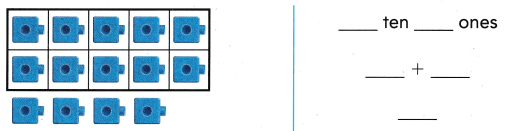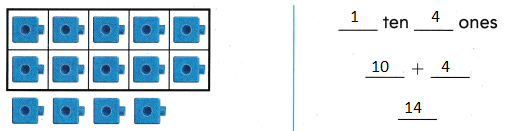Explanation:
10 + 4 = 14
1 tens and 4 ones
10 ones and 4 ones
This shows the 3 different ways

Question 2.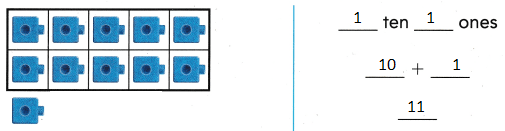Explanation:
10 + 4 = 11
1 tens and 1 ones
10 ones and 1 ones
This shows the 1 different ways

Problem Solving

Drawto show the number. Write the number different ways.

Question 3.
Anna has 8 apples. Jeremy has 7 apples. They put all their apples together. What number of apples did they make?____ ten ____ ones
___ + ____
______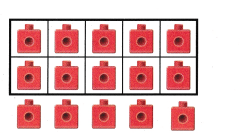1 tens and 5 ones
Explanation:
8 + 7 = 15
15 apples that they make

Texas Test Prep

Lesson Check

Question 4.
Myra drew 19 different stars on 12 pieces of paper. Which shows 19?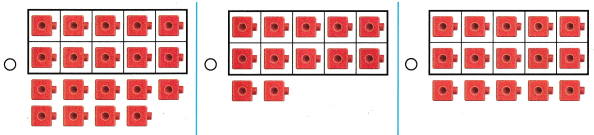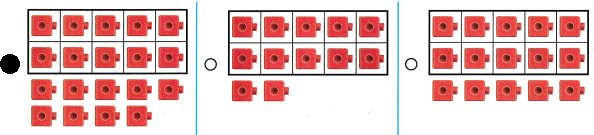Explanation:
Bubbled the correct option
1 tens and 9 ones

10 + 9 = 19

Question 5.
Jerry used counters to show 16. Which shows another way to write 16?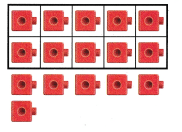(A) 1 ten 4 ones
(B) 6 tens 1 one
(C) 1 ten 6 ones
Explanation:
1 ten 6 ones
shows another way to write 16

Question 6.
Multi-Step Carla has 9 balls. Alex gives Carla 5 balls. Then he gives her 4 more balls. Which shows the number of balls Carla has?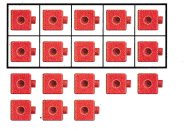(A) 1 ten 8 ones
(B) 1 ten 3 ones
(C) 1 ten 5 ones